Shortcuts

# Pearson’s Contingency Coefficient¶

## Module Interface¶

class torchmetrics.PearsonsContingencyCoefficient(num_classes, nan_strategy='replace', nan_replace_value=0.0, **kwargs)[source]

Compute Pearson’s Contingency Coefficient statistic measuring the association between two categorical (nominal) data series.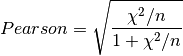where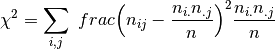where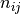denotes the number of times the values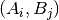are observed with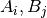represent frequencies of values in preds and target, respectively.

Pearson’s Contingency Coefficient is a symmetric coefficient, i.e.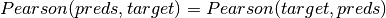.

The output values lies in [0, 1] with 1 meaning the perfect association.

Parameters
Returns

Pearson’s Contingency Coefficient statistic

Raises
• ValueError – If nan_strategy is not one of ‘replace’ and ‘drop’

• ValueError – If nan_strategy is equal to ‘replace’ and nan_replace_value is not an int or float

Example

>>> from torchmetrics import PearsonsContingencyCoefficient
>>> _ = torch.manual_seed(42)
>>> preds = torch.randint(0, 4, (100,))
>>> target = torch.round(preds + torch.randn(100)).clamp(0, 4)
>>> pearsons_contingency_coefficient = PearsonsContingencyCoefficient(num_classes=5)
>>> pearsons_contingency_coefficient(preds, target)
tensor(0.6948)


Initializes internal Module state, shared by both nn.Module and ScriptModule.

compute()[source]

Computer Pearson’s Contingency Coefficient statistic.

Return type

Tensor

update(preds, target)[source]

Update state with predictions and targets.

Parameters
• preds (Tensor) –

1D or 2D tensor of categorical (nominal) data:

• 1D shape: (batch_size,)

• 2D shape: (batch_size, num_classes)

• target (Tensor) –

1D or 2D tensor of categorical (nominal) data:

• 1D shape: (batch_size,)

• 2D shape: (batch_size, num_classes)

Return type

None

## Functional Interface¶

torchmetrics.functional.pearsons_contingency_coefficient(preds, target, nan_strategy='replace', nan_replace_value=0.0)[source]

Compute Pearson’s Contingency Coefficient measuring the association between two categorical (nominal) data series.wherewheredenotes the number of times the valuesare observed withrepresent frequencies of values in preds and target, respectively.

Pearson’s Contingency Coefficient is a symmetric coefficient, i.e..

The output values lies in [0, 1] with 1 meaning the perfect association.

Parameters
Return type

Tensor

Returns

Pearson’s Contingency Coefficient

Example

>>> from torchmetrics.functional import pearsons_contingency_coefficient
>>> _ = torch.manual_seed(42)
>>> preds = torch.randint(0, 4, (100,))
>>> target = torch.round(preds + torch.randn(100)).clamp(0, 4)
>>> pearsons_contingency_coefficient(preds, target)
tensor(0.6948)


### pearsons_contingency_coefficient_matrix¶

torchmetrics.functional.nominal.pearsons_contingency_coefficient_matrix(matrix, nan_strategy='replace', nan_replace_value=0.0)[source]

Compute Pearson’s Contingency Coefficient statistic between a set of multiple variables.

This can serve as a convenient tool to compute Pearson’s Contingency Coefficient for analyses of correlation between categorical variables in your dataset.

Parameters
Return type

Tensor

Returns

Pearson’s Contingency Coefficient statistic for a dataset of categorical variables

Example

>>> from torchmetrics.functional.nominal import pearsons_contingency_coefficient_matrix
>>> _ = torch.manual_seed(42)
>>> matrix = torch.randint(0, 4, (200, 5))
>>> pearsons_contingency_coefficient_matrix(matrix)
tensor([[1.0000, 0.2326, 0.1959, 0.2262, 0.2989],
[0.2326, 1.0000, 0.1386, 0.1895, 0.1329],
[0.1959, 0.1386, 1.0000, 0.1840, 0.2335],
[0.2262, 0.1895, 0.1840, 1.0000, 0.2737],
[0.2989, 0.1329, 0.2335, 0.2737, 1.0000]])


© Copyright Copyright (c) 2020-2023, Lightning-AI et al... Revision 6853c553.

Built with Sphinx using a theme provided by Read the Docs.
Versions
latest
stable
v0.11.2
v0.11.1
v0.11.0
v0.10.3
v0.10.2
v0.10.1
v0.10.0
v0.9.3
v0.9.2
v0.9.1
v0.9.0
v0.8.2
v0.8.1
v0.8.0
v0.7.3
v0.7.2
v0.7.1
v0.7.0
v0.6.2
v0.6.1
v0.6.0
v0.5.1
v0.5.0
v0.4.1
v0.4.0
v0.3.2
v0.3.1
v0.3.0
v0.2.0
v0.1.0